# How might you split the array How might

• Slides: 28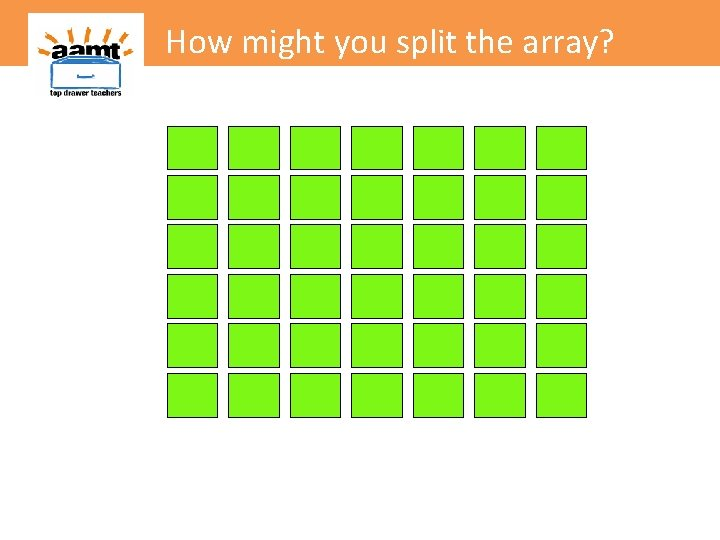How might you split the array?How might you split the array?How might you split the array? I know 6 rows of 5 is 30.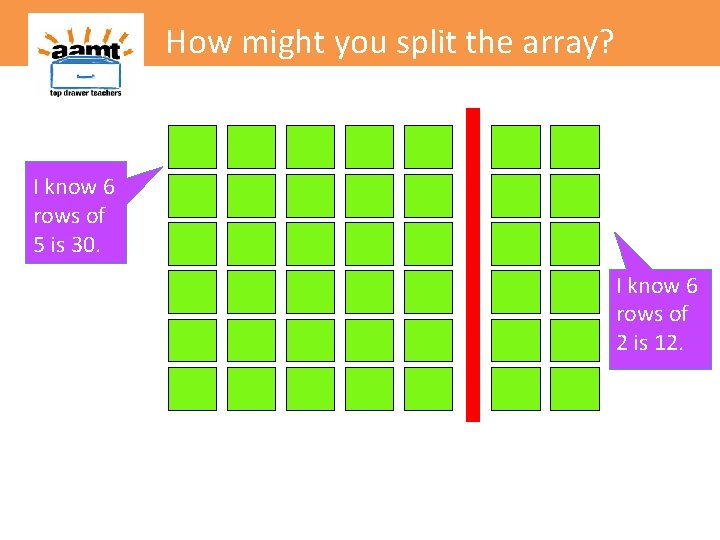How might you split the array? I know 6 rows of 5 is 30. I know 6 rows of 2 is 12.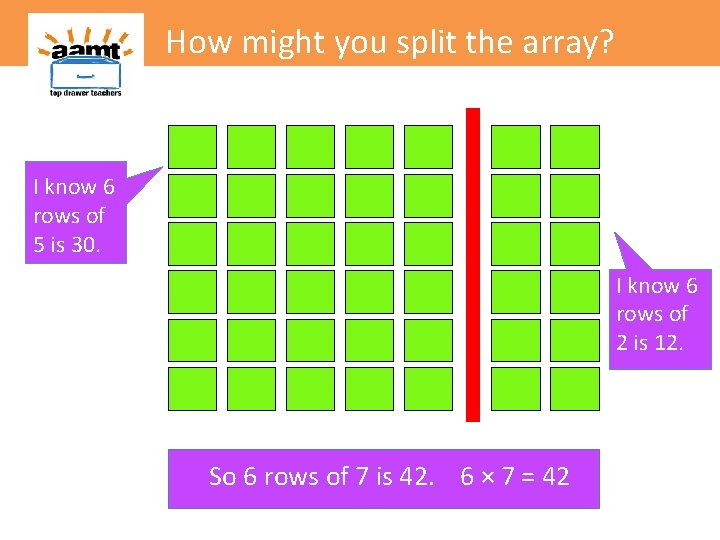How might you split the array? I know 6 rows of 5 is 30. I know 6 rows of 2 is 12. So 6 rows of 7 is 42. 6 × 7 = 42How might you split the array? Is there another way?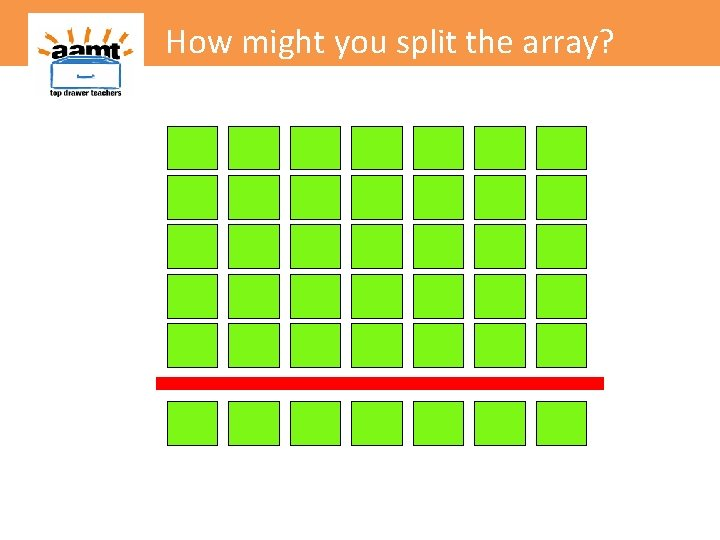How might you split the array? I know! 6 rows of 5 is 30How might you split the array? I know 5 rows of 7 is 35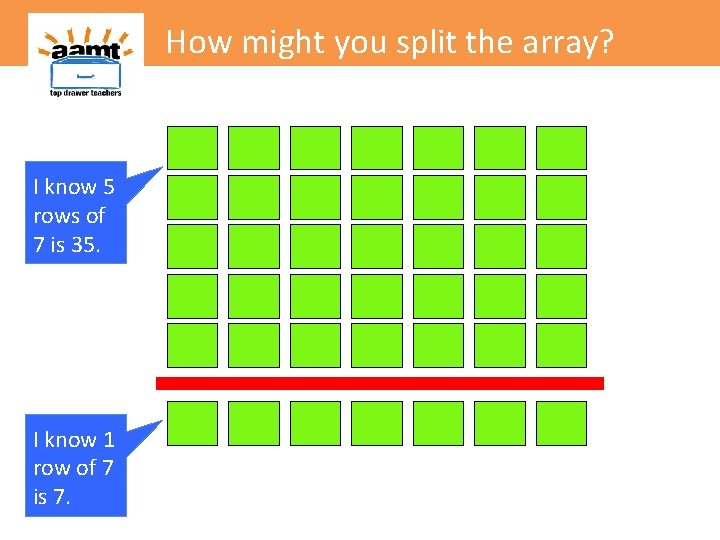How might you split the array? I know 5 rows of 7 is 35 I know 1 row of 7 is 7.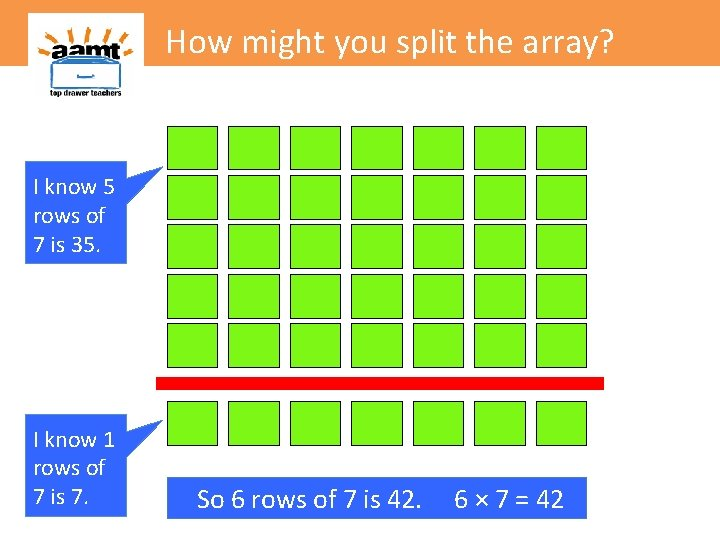How might you split the array? I know 5 rows of 7 is 35 I know 1 rows of 7 is 7. So 6 rows of 7 is 42. 6 × 7 = 42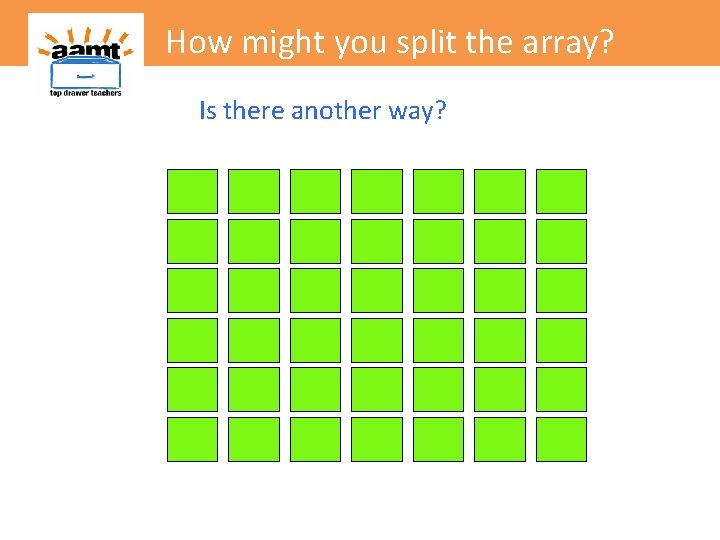How might you split the array? Is there another way?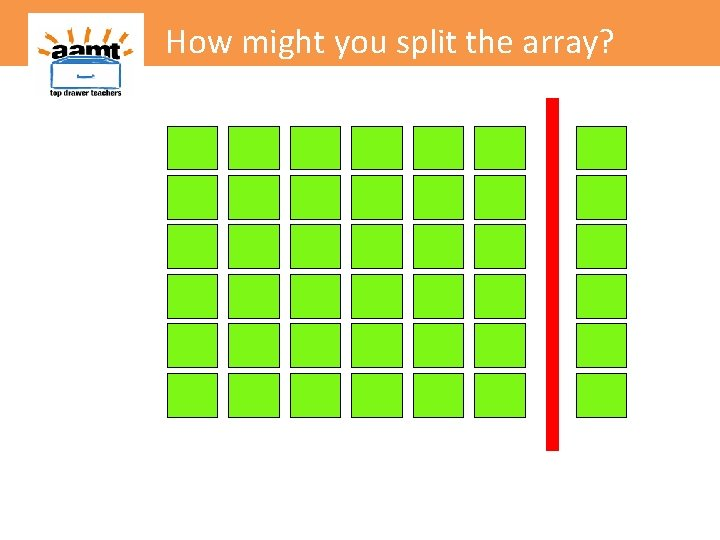How might you split the array?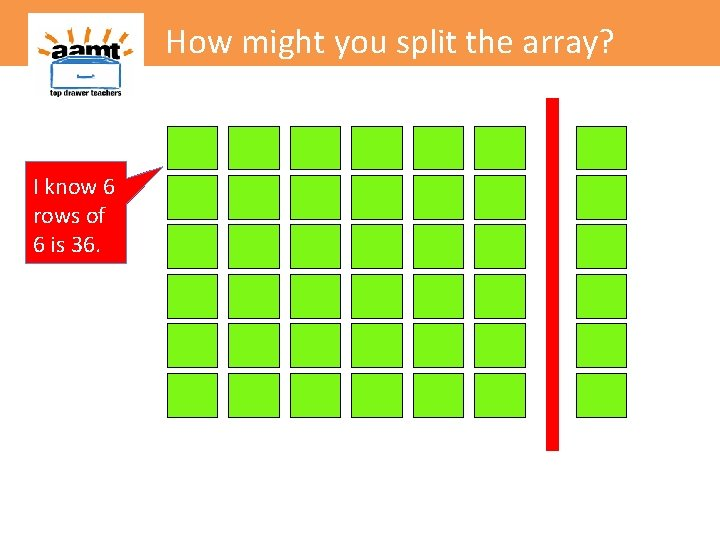How might you split the array? I know 6 rows of 6 is 36.How might you split the array? I know 6 rows of 6 is 36. I know 6 rows of 1 is 6.How might you split the array? I know 6 rows of 6 is 36. So 6 rows of 7 is 42. 6 × 7 = 42 I know 6 rows of 1 is 6.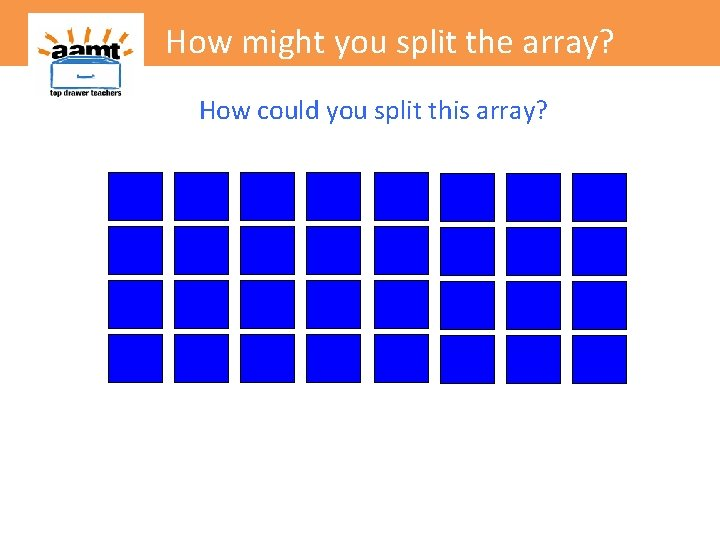How might you split the array? How could you split this array?How might you split the array? 4 rows of 5 4 × 5 = 20 4 rows of 3 4 × 3 = 12How might you split the array? 4 rows of 5 4 × 5 = 20 4 rows of 8 4 × 8 = 32 4 rows of 3 4 × 3 = 12How might you split the array? Is there another way?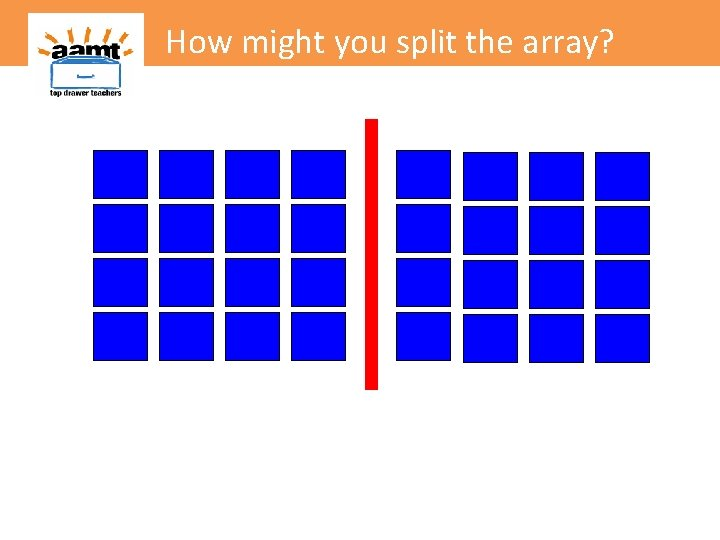How might you split the array?How might you split the array? 4 rows of 4 4 × 4 = 16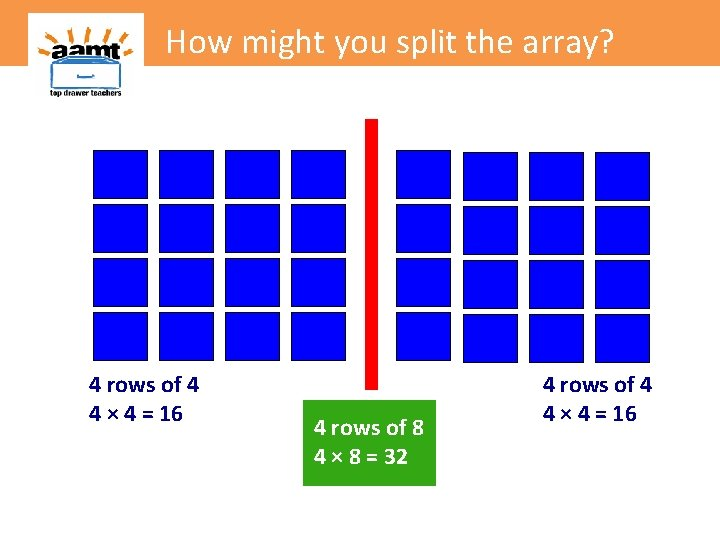How might you split the array? 4 rows of 4 4 × 4 = 16 4 rows of 8 4 × 8 = 32 4 rows of 4 4 × 4 = 16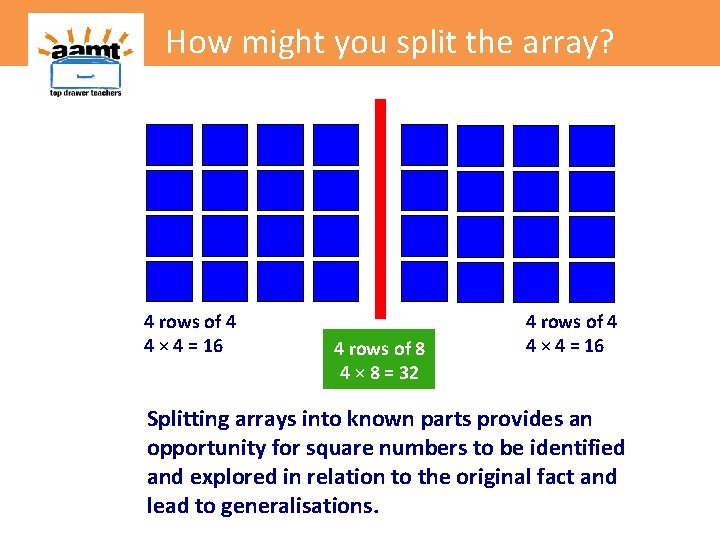How might you split the array? 4 rows of 4 4 × 4 = 16 4 rows of 8 4 × 8 = 32 4 rows of 4 4 × 4 = 16 Splitting arrays into known parts provides an opportunity for square numbers to be identified and explored in relation to the original fact and lead to generalisations.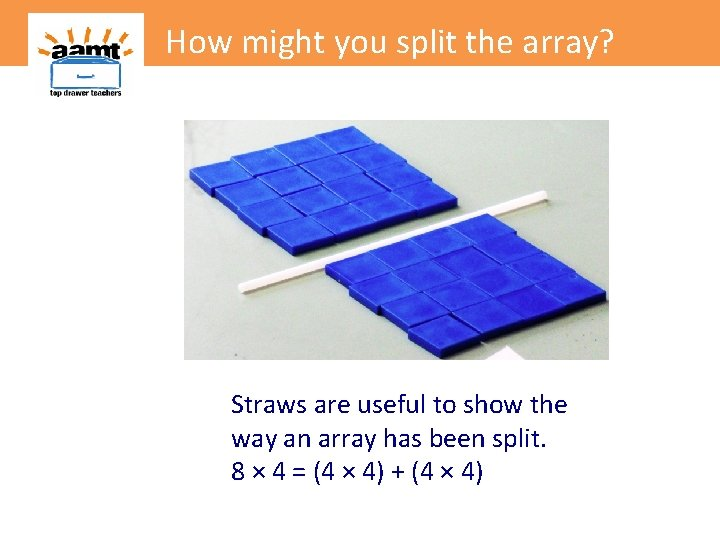How might you split the array? Straws are useful to show the way an array has been split. 8 × 4 = (4 × 4) + (4 × 4)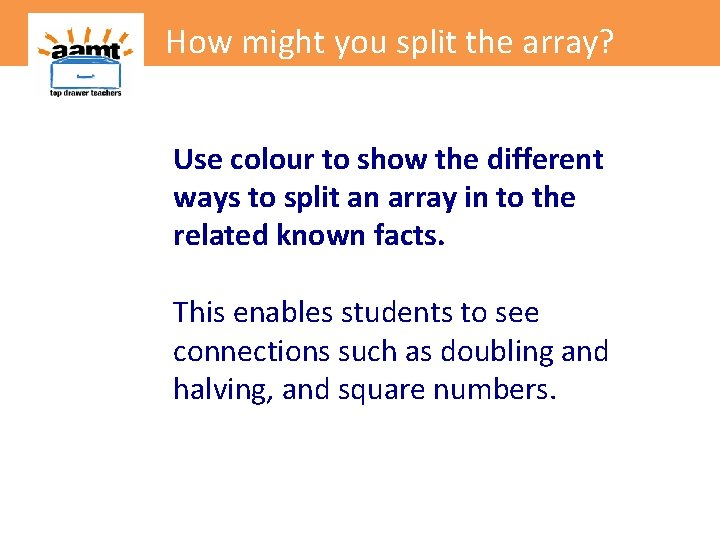How might you split the array? Use colour to show the different ways to split an array in to the related known facts. This enables students to see connections such as doubling and halving, and square numbers.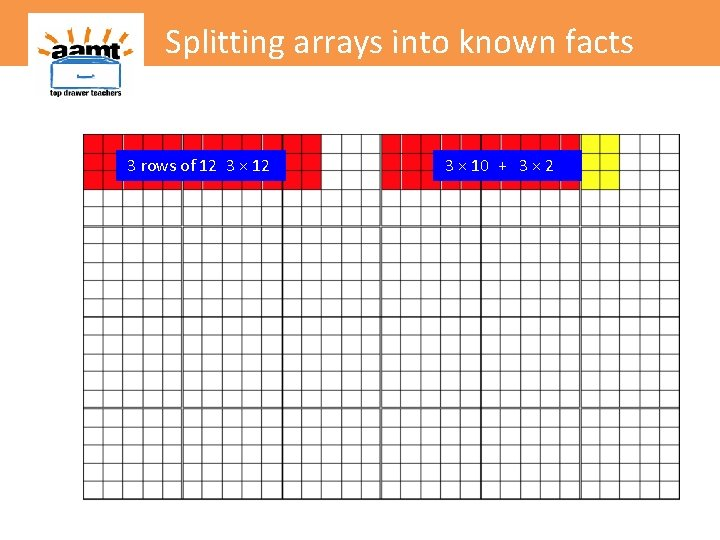Splitting arrays into known facts 3 rows of 12 3 × 10 + 3 × 2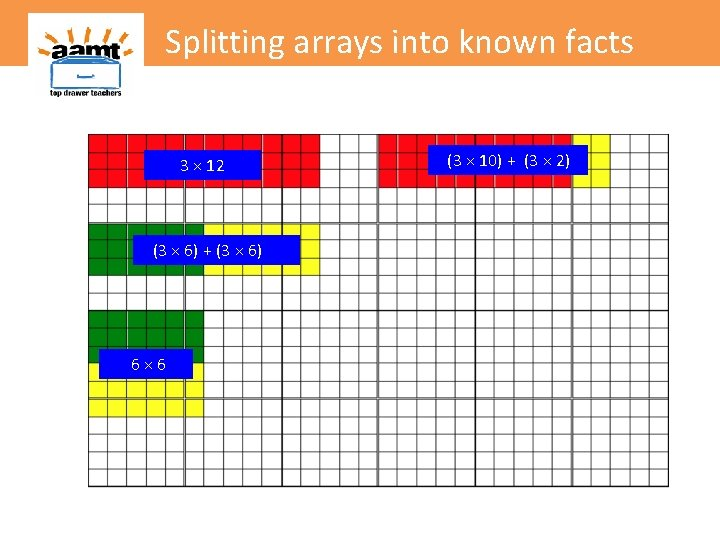Splitting arrays into known facts 3 × 12 (3 × 6) + (3 × 6) 6× 6 (3 × 10) + (3 × 2)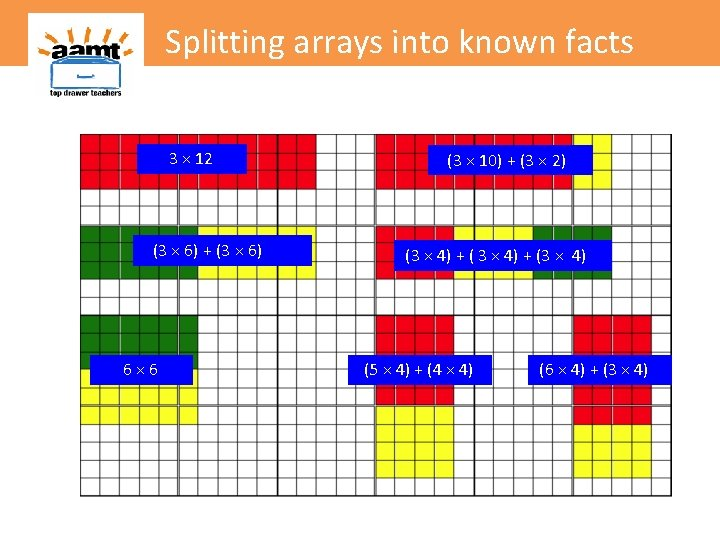Splitting arrays into known facts 3 × 12 (3 × 6) + (3 × 6) 6× 6 (3 × 10) + (3 × 2) (3 × 4) + (3 × 4) (5 × 4) + (4 × 4) (6 × 4) + (3 × 4)